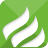# International packet

If the weight is less than 2kg, the sum of length, width and height of the outer package is less than 90cm, and the longest side is less than 60cm...If the weight is less than 2kg, the sum of length, width and height of the outer package is less than 90cm, and the longest side is less than 60cm...If the weight is less than 2kg, the sum of length, width and height of the outer package is less than 90cm, and the longest side is less than 60cm...If the weight is less than 2kg, the sum of length, width and height of the outer package is less than 90cm, and the longest side is less than 60cm...

0.0533s